# 04 - MSM analysis¶In this notebook, we will cover how to analyze an MSM and how the modeled processes correspond to MSM spectral properties. We assume that you are familiar with data loading/visualization (Notebook 01 ➜ 📓), dimension reduction (Notebook 02 ➜ 📓), and the estimation and validation process (Notebook 03 ➜ 📓).

Maintainers: [@cwehmeyer](https://github.com/cwehmeyer), [@marscher](https://github.com/marscher), [@thempel](https://github.com/thempel), [@psolsson](https://github.com/psolsson)

Remember: - to run the currently highlighted cell, hold ⇧ Shift and press ⏎ Enter; - to get help for a specific function, place the cursor within the function’s brackets, hold ⇧ Shift, and press ⇥ Tab; - you can find the full documentation at PyEMMA.org.

:

%matplotlib inline
import matplotlib.pyplot as plt
import matplotlib as mpl
import numpy as np
import mdshare
import pyemma

/storage/mi/marscher/software/miniconda3/conda-bld/pyemma-doc_1607519340854/_h_env_placehold_placehold_placehold_placehold_placehold_placehold_placehold_placehold_placehold_placehold_placehold_placehold_placehold_placehold_placehold_placehold_placehold_pl/lib/python3.9/site-packages/mdshare/repository.py:53: YAMLLoadWarning: calling yaml.load() without Loader=... is deprecated, as the default Loader is unsafe. Please read https://msg.pyyaml.org/load for full details.


## Case 1: preprocessed, two-dimensional data (toy model)¶

We load the two-dimensional trajectory from an archive using numpy, directly discretize the full space using $$k$$-means clustering, visualize the marginal and joint distributions of both components as well as the cluster centers, and show the implied timescale (ITS) convergence:

:

file = mdshare.fetch('hmm-doublewell-2d-100k.npz', working_directory='data')
data = fh['trajectory']

cluster = pyemma.coordinates.cluster_kmeans(data, k=50, max_iter=50)
its = pyemma.msm.its(
cluster.dtrajs, lags=[1, 2, 3, 5, 7, 10], nits=3, errors='bayes')

fig, axes = plt.subplots(1, 3, figsize=(12, 3))
pyemma.plots.plot_feature_histograms(data, feature_labels=['$x$', '$y$'], ax=axes)
pyemma.plots.plot_density(*data.T, ax=axes, cbar=False, alpha=0.1)
axes.scatter(*cluster.clustercenters.T, s=15, c='C1')
axes.set_xlabel('$x$')
axes.set_ylabel('$y$')
axes.set_xlim(-4, 4)
axes.set_ylim(-4, 4)
axes.set_aspect('equal')
pyemma.plots.plot_implied_timescales(its, ylog=False, ax=axes)
fig.tight_layout()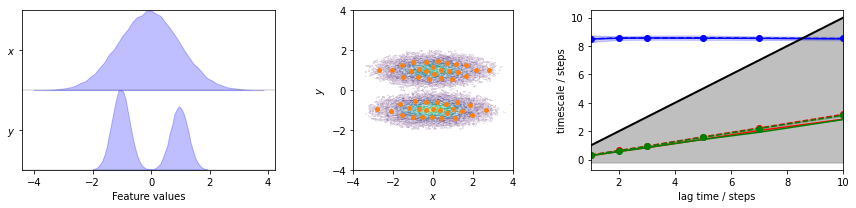The plots show us the marginal (left panel) and joint distributions along with the cluster centers (middle panel). The implied timescales are converged (right panel).

Before we proceed, let’s have a look at the implied timescales error bars. They were computed from a Bayesian MSM, as requested by the errors='bayes' argument of the pyemma.msm.its() function. As mentioned before, Bayesian MSMs incorporate a sample of transition matrices. Target properties such as implied timescales can now simply be computed from the individual matrices. Thereby, the posterior distributions of these properties can be estimated. The ITS plot shows a confidence interval that contains $$95\%$$ of the Bayesian samples.

:

bayesian_msm = pyemma.msm.bayesian_markov_model(cluster.dtrajs, lag=1, conf=0.95)


For any PyEMMA method that derives target properties from MSMs, sample mean and confidence intervals (as defined by the function argument above) are directly accessible with sample_mean() and sample_conf(). Further, sample_std() is available for computing the standard deviation. In the more general case, it might be interesting to extract the full sample of a function evaluation with sample_f(). The syntax is equivalent for all those functions.

:

sample_mean = bayesian_msm.sample_mean('timescales', k=1)
sample_conf_l, sample_conf_r = bayesian_msm.sample_conf('timescales', k=1)

print('Mean of first ITS: {:f}'.format(sample_mean))
print('Confidence interval: [{:f}, {:f}]'.format(sample_conf_l, sample_conf_r))

Mean of first ITS: 8.515907
Confidence interval: [8.293750, 8.741939]


Please note that sample mean and maximum likelihood estimates are not identical and generally do not provide numerically identical results.

Now, for the sake of simplicity we proceed with the analysis of a maximum likelihood MSM. We estimate it at lag time $$1$$ step…

:

msm = pyemma.msm.estimate_markov_model(cluster.dtrajs, lag=1)


… and check for disconnectivity. The MSM is constructed on the largest set of discrete states that are (reversibly) connected. The active_state_fraction and active_count_fraction show us the fraction of discrete states and transition counts from our data which are part of this largest set and, thus, used for the model:

:

print('fraction of states used = {:f}'.format(msm.active_state_fraction))
print('fraction of counts used = {:f}'.format(msm.active_count_fraction))

fraction of states used = 1.000000
fraction of counts used = 1.000000


The fraction is, in both cases, $$1$$ and, thus, we have no disconnected states (which we would have to exclude from our analysis).

If there were any disconnectivities in our data (fractions $$<1$$), we could access the indices of the active states (members of the largest connected set) via the active_set attribute:

:

print(msm.active_set)

[ 0  1  2  3  4  5  6  7  8  9 10 11 12 13 14 15 16 17 18 19 20 21 22 23
24 25 26 27 28 29 30 31 32 33 34 35 36 37 38 39 40 41 42 43 44 45 46 47
48 49]


With this potential issue out of the way, we can extract our first (stationary/thermodynamic) property, the stationary_distribution or, as a shortcut, pi:

:

print(msm.stationary_distribution)
print('sum of weights = {:f}'.format(msm.pi.sum()))

[0.00774006 0.02036021 0.03419033 0.01088011 0.02615027 0.02860024
0.01689018 0.01344014 0.02545027 0.03679024 0.02284023 0.01464016
0.03531042 0.02236026 0.0202502  0.02386024 0.01884018 0.00500001
0.00951009 0.02290024 0.01260013 0.00502005 0.01483014 0.0266203
0.01825015 0.02742029 0.01547016 0.01337016 0.03739042 0.02876032
0.02986032 0.01565019 0.01925013 0.01182009 0.01654017 0.01261013
0.02655025 0.01865018 0.01701017 0.01711015 0.02078018 0.02949028
0.02859029 0.01710018 0.0217802  0.02548028 0.01292013 0.00348004
0.02549028 0.01410013]
sum of weights = 1.000000


The attribute msm.pi tells us, for each discrete state, the absolute probability of observing said state in global equilibrium. Mathematically speaking, the stationary distribution $$\pi$$ is the left eigenvector of the transition matrix $$\mathbf{P}$$ to the eigenvalue $$1$$:

$\pi^\top \mathbf{P} = \pi^\top.$

Please note that the $$\pi$$ is fundamentaly different from a normalized histogram of states: for the histogram of states to accurately describe the stationary distribution, the data needs to be sampled from global equilibrium, i.e, the data points need to be statistically independent. The MSM approach, on the other hand, only requires local equilibrium, i.e., statistical independence of state transitions. Thus, the MSM approach requires a much weaker and, in practice, much easier to satisfy condition than simply counting state visits.

We can use the stationary distribution to, e.g., visualize the weight of the dicrete states and, thus, to highlight which areas of our feature space are most probable. Here, we show all data points in a two dimensional scatter plot and color/weight them according to their discrete state membership:

:

fig, ax, misc = pyemma.plots.plot_contour(
*data.T, msm.pi[cluster.dtrajs],
cbar_label='stationary distribution',
ax.scatter(*cluster.clustercenters.T, s=15, c='C1')
ax.set_xlabel('$x$')
ax.set_ylabel('$y$')
ax.set_xlim(-4, 4)
ax.set_ylim(-4, 4)
ax.set_aspect('equal')
fig.tight_layout()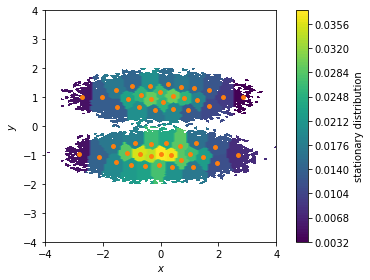The stationary distribution can also be used to correct the pyemma.plots.plot_free_energy() function that we used to visualize this dataset in Notebook 01 ➜ 📓. This might be necessary if the data points are not sampled from global equilibrium.

In this case, we assign the weight of the corresponding discrete state to each data point and pass this information to the plotting function via its weights parameter:

:

fig, ax, misc = pyemma.plots.plot_free_energy(
*data.T,
weights=np.concatenate(msm.trajectory_weights()),
legacy=False)
ax.set_xlabel('$x$')
ax.set_ylabel('$y$')
ax.set_xlim(-4, 4)
ax.set_ylim(-4, 4)
ax.set_aspect('equal')
fig.tight_layout()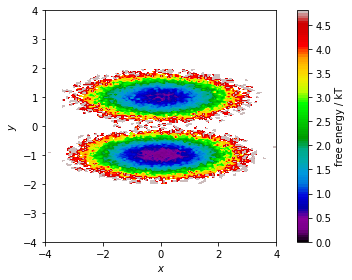We will see further uses of the stationary distribution later. But for now, we continue the analysis of our model by visualizing its (right) eigenvectors which encode the dynamical processes. First, we notice that the first right eigenvector is a constant $$1$$.

:

eigvec = msm.eigenvectors_right()
print('first eigenvector is one: {} (min={}, max={})'.format(
np.allclose(eigvec[:, 0], 1, atol=1e-15), eigvec[:, 0].min(), eigvec[:, 0].max()))

first eigenvector is one: True (min=0.9999999999999928, max=1.0000000000000027)


Second, the higher eigenvectors can be visualized as follows:

:

fig, axes = plt.subplots(1, 3, figsize=(12, 3))
for i, ax in enumerate(axes.flat):
pyemma.plots.plot_contour(
*data.T, eigvec[cluster.dtrajs, i + 1], ax=ax, cmap='PiYG',
cbar_label='{}. right eigenvector'.format(i + 2), mask=True)
ax.scatter(*cluster.clustercenters.T, s=15, c='C1')
ax.set_xlabel('$x$')
ax.set_xlim(-4, 4)
ax.set_ylim(-4, 4)
ax.set_aspect('equal')
axes.set_ylabel('$y$')
fig.tight_layout()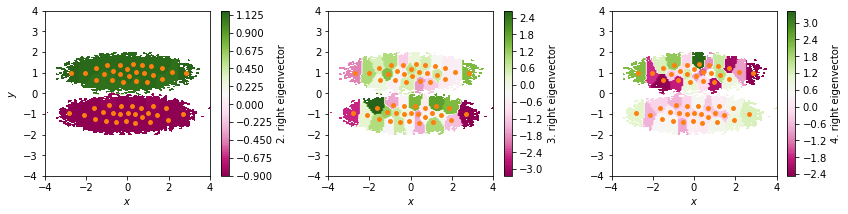The right eigenvectors can be used to visualize the processes governed by the corresponding implied timescales. The first right eigenvector (always) is $$(1,\dots,1)^\top$$ for an MSM transition matrix and it corresponds to the stationary process (infinite implied timescale).

The second right eigenvector corresponds to the slowest process; its entries are negative for one group of discrete states and positive for the other group. This tells us that the slowest process happens between these two groups and that the process relaxes on the slowest ITS ($$\approx 8.5$$ steps).

The third and fourth eigenvectors show a larger spread of values and no clear grouping. In combination with the ITS convergence plot, we can safely assume that these eigenvectors contain just noise and do not indicate any resolved processes.

We then continue to validate our MSM with a CK test for $$2$$ metastable states which are already indicated by the second right eigenvector.

:

nstates = 2
pyemma.plots.plot_cktest(msm.cktest(nstates));

:

(<Figure size 432x432 with 4 Axes>,
array([[<AxesSubplot:ylabel='probability'>, <AxesSubplot:>],
[<AxesSubplot:xlabel='lag time (steps)', ylabel='probability'>,
<AxesSubplot:xlabel='lag time (steps)'>]], dtype=object))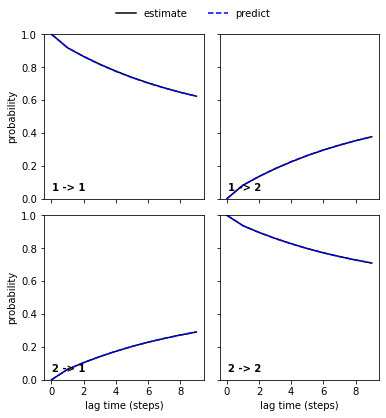We now save the model to do more analyses with PCCA++ and TPT in Notebook 05 ➜ 📓:

:

cluster.save('nb4.pyemma', model_name='doublewell_cluster', overwrite=True)
msm.save('nb4.pyemma', model_name='doublewell_msm', overwrite=True)
bayesian_msm.save('nb4.pyemma', model_name='doublewell_bayesian_msm', overwrite=True)

---------------------------------------------------------------------------
OSError                                   Traceback (most recent call last)
<ipython-input-1-afd58d4fe75b> in <module>
----> 1 cluster.save('nb4.pyemma', model_name='doublewell_cluster', overwrite=True)
2 msm.save('nb4.pyemma', model_name='doublewell_msm', overwrite=True)
3 bayesian_msm.save('nb4.pyemma', model_name='doublewell_bayesian_msm', overwrite=True)

/storage/mi/marscher/software/miniconda3/conda-bld/pyemma-doc_1607519340854/_h_env_placehold_placehold_placehold_placehold_placehold_placehold_placehold_placehold_placehold_placehold_placehold_placehold_placehold_placehold_placehold_placehold_placehold_pl/lib/python3.9/site-packages/pyemma/_base/serialization/serialization.py in save(self, file_name, model_name, overwrite, save_streaming_chain)
236         >>> np.testing.assert_equal(m.P, inst_restored.P) # doctest: +SKIP
237         """
--> 238         from pyemma._base.serialization.h5file import H5File
239         try:
240             with H5File(file_name=file_name, mode='a') as f:

/storage/mi/marscher/software/miniconda3/conda-bld/pyemma-doc_1607519340854/_h_env_placehold_placehold_placehold_placehold_placehold_placehold_placehold_placehold_placehold_placehold_placehold_placehold_placehold_placehold_placehold_placehold_placehold_pl/lib/python3.9/site-packages/pyemma/_base/serialization/h5file.py in <module>
20 import numpy as np
21 from io import BytesIO
---> 22 from .pickle_extensions import HDF5PersistentPickler
23
24 __author__ = 'marscher'

/storage/mi/marscher/software/miniconda3/conda-bld/pyemma-doc_1607519340854/_h_env_placehold_placehold_placehold_placehold_placehold_placehold_placehold_placehold_placehold_placehold_placehold_placehold_placehold_placehold_placehold_placehold_placehold_pl/lib/python3.9/site-packages/pyemma/_base/serialization/pickle_extensions.py in <module>
66
67 # we cache this during runtime
---> 68 _DEFAULT_BLOSC_OPTIONS = _check_blosc_avail()
69
70

/storage/mi/marscher/software/miniconda3/conda-bld/pyemma-doc_1607519340854/_h_env_placehold_placehold_placehold_placehold_placehold_placehold_placehold_placehold_placehold_placehold_placehold_placehold_placehold_placehold_placehold_placehold_placehold_pl/lib/python3.9/site-packages/pyemma/_base/serialization/pickle_extensions.py in _check_blosc_avail()
44     fid, name = tempfile.mkstemp()
45     try:
---> 46         with h5py.File(name) as h5f:
47             try:
48                 h5f.create_dataset('test', shape=(1,1), **blosc_opts)

/storage/mi/marscher/software/miniconda3/conda-bld/pyemma-doc_1607519340854/_h_env_placehold_placehold_placehold_placehold_placehold_placehold_placehold_placehold_placehold_placehold_placehold_placehold_placehold_placehold_placehold_placehold_placehold_pl/lib/python3.9/site-packages/h5py/_hl/files.py in __init__(self, name, mode, driver, libver, userblock_size, swmr, rdcc_nslots, rdcc_nbytes, rdcc_w0, track_order, fs_strategy, fs_persist, fs_threshold, **kwds)
422             with phil:
423                 fapl = make_fapl(driver, libver, rdcc_nslots, rdcc_nbytes, rdcc_w0, **kwds)
--> 424                 fid = make_fid(name, mode, userblock_size,
425                                fapl, fcpl=make_fcpl(track_order=track_order, fs_strategy=fs_strategy,
426                                fs_persist=fs_persist, fs_threshold=fs_threshold),

/storage/mi/marscher/software/miniconda3/conda-bld/pyemma-doc_1607519340854/_h_env_placehold_placehold_placehold_placehold_placehold_placehold_placehold_placehold_placehold_placehold_placehold_placehold_placehold_placehold_placehold_placehold_placehold_pl/lib/python3.9/site-packages/h5py/_hl/files.py in make_fid(name, mode, userblock_size, fapl, fcpl, swmr)
188         if swmr and swmr_support:
--> 190         fid = h5f.open(name, flags, fapl=fapl)
191     elif mode == 'r+':
192         fid = h5f.open(name, h5f.ACC_RDWR, fapl=fapl)

h5py/_objects.pyx in h5py._objects.with_phil.wrapper()

h5py/_objects.pyx in h5py._objects.with_phil.wrapper()

h5py/h5f.pyx in h5py.h5f.open()



## Case 2: low-dimensional molecular dynamics data (alanine dipeptide)¶

We fetch the alanine dipeptide data set, load the backbone torsions into memory, directly discretize the full space using $$k$$-means clustering, visualize the margial and joint distributions of both components as well as the cluster centers, and show the ITS convergence to help selecting a suitable lag time:

:

pdb = mdshare.fetch('alanine-dipeptide-nowater.pdb', working_directory='data')
files = mdshare.fetch('alanine-dipeptide-*-250ns-nowater.xtc', working_directory='data')

feat = pyemma.coordinates.featurizer(pdb)
data_concatenated = np.concatenate(data)

cluster = pyemma.coordinates.cluster_kmeans(data, k=100, max_iter=50, stride=10)
dtrajs_concatenated = np.concatenate(cluster.dtrajs)

its = pyemma.msm.its(
cluster.dtrajs, lags=[1, 2, 5, 10, 20, 50], nits=4, errors='bayes')

fig, axes = plt.subplots(1, 3, figsize=(12, 3))
pyemma.plots.plot_feature_histograms(
np.concatenate(data), feature_labels=['$\Phi$', '$\Psi$'], ax=axes)
pyemma.plots.plot_density(*data_concatenated.T, ax=axes, cbar=False, alpha=0.1)
axes.scatter(*cluster.clustercenters.T, s=15, c='C1')
axes.set_xlabel('$\Phi$')
axes.set_ylabel('$\Psi$')
pyemma.plots.plot_implied_timescales(its, ax=axes, units='ps')
fig.tight_layout()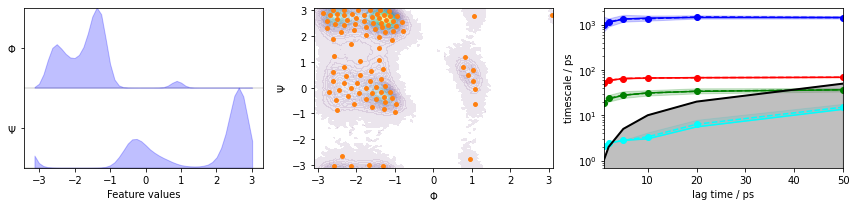The plots show us the marginal (left panel) and joint distributions along with the cluster centers (middle panel). The implied timescales are converged (right panel).

We then estimate an MSM at lag time $$10$$ ps and visualize the stationary distribution by coloring all data points according to the stationary weight of the discrete state they belong to:

:

msm = pyemma.msm.estimate_markov_model(cluster.dtrajs, lag=10, dt_traj='1 ps')

print('fraction of states used = {:f}'.format(msm.active_state_fraction))
print('fraction of counts used = {:f}'.format(msm.active_count_fraction))

fig, ax, misc = pyemma.plots.plot_contour(
*data_concatenated.T, msm.pi[dtrajs_concatenated],
cbar_label='stationary_distribution',
ax.scatter(*cluster.clustercenters.T, s=15, c='C1')
ax.set_xlabel('$\Phi$')
ax.set_ylabel('$\Psi$')
fig.tight_layout()

fraction of states used = 1.000000
fraction of counts used = 1.000000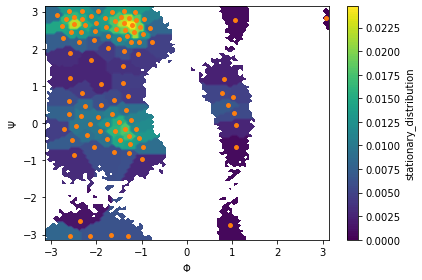Next, we visualize the first six right eigenvectors:

:

eigvec = msm.eigenvectors_right()
print('first eigenvector is one: {} (min={}, max={})'.format(
np.allclose(eigvec[:, 0], 1, atol=1e-15), eigvec[:, 0].min(), eigvec[:, 0].max()))

fig, axes = plt.subplots(2, 3, figsize=(12, 6))
for i, ax in enumerate(axes.flat):
pyemma.plots.plot_contour(
*data_concatenated.T, eigvec[dtrajs_concatenated, i + 1], ax=ax, cmap='PiYG',
cbar_label='{}. right eigenvector'.format(i + 2), mask=True)
ax.scatter(*cluster.clustercenters.T, s=15, c='C1')
ax.set_xlabel('$\Phi$')
ax.set_ylabel('$\Psi$')
fig.tight_layout()

first eigenvector is one: True (min=0.9999999999999793, max=1.0000000000003757)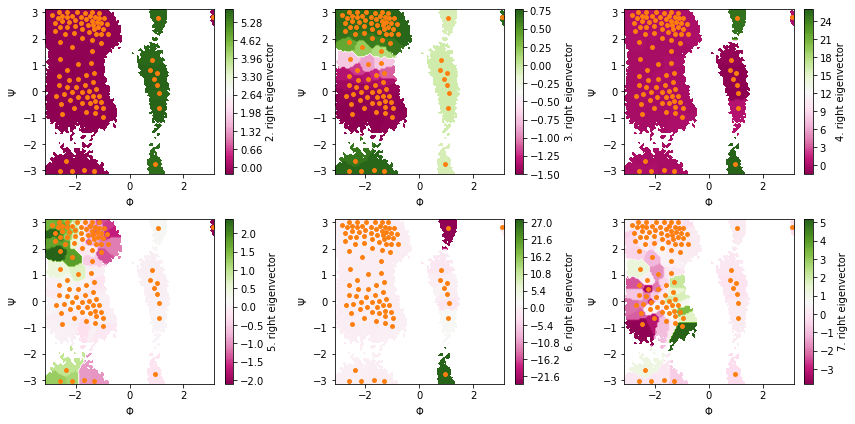Again, we have the $$(1,\dots,1)^\top$$ first right eigenvector of the stationary process.

The second to fourth right eigenvectors illustrate the three slowest processes which are (in that order):

• rotation of the $$\Phi$$ dihedral,

• rotation of the $$\Psi$$ dihedral when $$\Phi\approx-2$$ rad, and

• rotation of the $$\Psi$$ dihedral when $$\Phi\approx1$$ rad.

Eigenvectors five, six, and seven indicate further processes which, however, relax faster than the lag time and cannot be resolved clearly.

We now proceed our validation process using a Bayesian MSM with four metastable states:

:

nstates = 4
bayesian_msm = pyemma.msm.bayesian_markov_model(cluster.dtrajs, lag=10, dt_traj='1 ps')
pyemma.plots.plot_cktest(bayesian_msm.cktest(nstates), units='ps');

:

(<Figure size 720x720 with 16 Axes>,
array([[<AxesSubplot:ylabel='probability'>, <AxesSubplot:>,
<AxesSubplot:>, <AxesSubplot:>],
[<AxesSubplot:ylabel='probability'>, <AxesSubplot:>,
<AxesSubplot:>, <AxesSubplot:>],
[<AxesSubplot:ylabel='probability'>, <AxesSubplot:>,
<AxesSubplot:>, <AxesSubplot:>],
[<AxesSubplot:xlabel='lag time (ps)', ylabel='probability'>,
<AxesSubplot:xlabel='lag time (ps)'>,
<AxesSubplot:xlabel='lag time (ps)'>,
<AxesSubplot:xlabel='lag time (ps)'>]], dtype=object))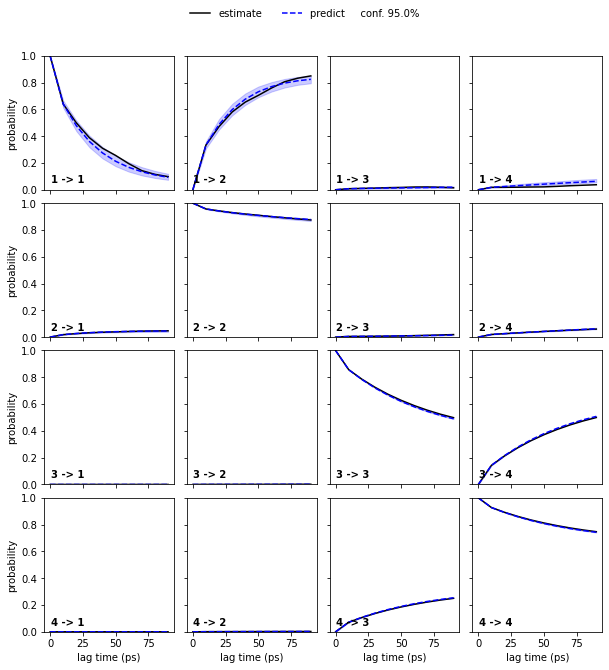We note that four metastable states are a reasonable choice for our MSM.

In general, the number of metastable states is a modeler’s choice; it is adjusted to map the kinetics to be modeled. In the current example, increasing the resolution with a higher number of metastable states or resolving only the slowest process between $$2$$ states would be possible. However, the number of states is not arbitrary as the observed processes in metastable state space need not be Markovian in general. A failed Chapman-Kolmogorov test can thus also hint to a bad choice of the metastable state number.

In order to perform further analysis, we save the model to disk:

:

cluster.save('nb4.pyemma', model_name='ala2_cluster', overwrite=True)
msm.save('nb4.pyemma', model_name='ala2_msm', overwrite=True)
bayesian_msm.save('nb4.pyemma', model_name='ala2_bayesian_msm', overwrite=True)

---------------------------------------------------------------------------
OSError                                   Traceback (most recent call last)
<ipython-input-1-a197c32106d4> in <module>
----> 1 cluster.save('nb4.pyemma', model_name='ala2_cluster', overwrite=True)
2 msm.save('nb4.pyemma', model_name='ala2_msm', overwrite=True)
3 bayesian_msm.save('nb4.pyemma', model_name='ala2_bayesian_msm', overwrite=True)

/storage/mi/marscher/software/miniconda3/conda-bld/pyemma-doc_1607519340854/_h_env_placehold_placehold_placehold_placehold_placehold_placehold_placehold_placehold_placehold_placehold_placehold_placehold_placehold_placehold_placehold_placehold_placehold_pl/lib/python3.9/site-packages/pyemma/_base/serialization/serialization.py in save(self, file_name, model_name, overwrite, save_streaming_chain)
236         >>> np.testing.assert_equal(m.P, inst_restored.P) # doctest: +SKIP
237         """
--> 238         from pyemma._base.serialization.h5file import H5File
239         try:
240             with H5File(file_name=file_name, mode='a') as f:

/storage/mi/marscher/software/miniconda3/conda-bld/pyemma-doc_1607519340854/_h_env_placehold_placehold_placehold_placehold_placehold_placehold_placehold_placehold_placehold_placehold_placehold_placehold_placehold_placehold_placehold_placehold_placehold_pl/lib/python3.9/site-packages/pyemma/_base/serialization/h5file.py in <module>
20 import numpy as np
21 from io import BytesIO
---> 22 from .pickle_extensions import HDF5PersistentPickler
23
24 __author__ = 'marscher'

/storage/mi/marscher/software/miniconda3/conda-bld/pyemma-doc_1607519340854/_h_env_placehold_placehold_placehold_placehold_placehold_placehold_placehold_placehold_placehold_placehold_placehold_placehold_placehold_placehold_placehold_placehold_placehold_pl/lib/python3.9/site-packages/pyemma/_base/serialization/pickle_extensions.py in <module>
66
67 # we cache this during runtime
---> 68 _DEFAULT_BLOSC_OPTIONS = _check_blosc_avail()
69
70

/storage/mi/marscher/software/miniconda3/conda-bld/pyemma-doc_1607519340854/_h_env_placehold_placehold_placehold_placehold_placehold_placehold_placehold_placehold_placehold_placehold_placehold_placehold_placehold_placehold_placehold_placehold_placehold_pl/lib/python3.9/site-packages/pyemma/_base/serialization/pickle_extensions.py in _check_blosc_avail()
44     fid, name = tempfile.mkstemp()
45     try:
---> 46         with h5py.File(name) as h5f:
47             try:
48                 h5f.create_dataset('test', shape=(1,1), **blosc_opts)

/storage/mi/marscher/software/miniconda3/conda-bld/pyemma-doc_1607519340854/_h_env_placehold_placehold_placehold_placehold_placehold_placehold_placehold_placehold_placehold_placehold_placehold_placehold_placehold_placehold_placehold_placehold_placehold_pl/lib/python3.9/site-packages/h5py/_hl/files.py in __init__(self, name, mode, driver, libver, userblock_size, swmr, rdcc_nslots, rdcc_nbytes, rdcc_w0, track_order, fs_strategy, fs_persist, fs_threshold, **kwds)
422             with phil:
423                 fapl = make_fapl(driver, libver, rdcc_nslots, rdcc_nbytes, rdcc_w0, **kwds)
--> 424                 fid = make_fid(name, mode, userblock_size,
425                                fapl, fcpl=make_fcpl(track_order=track_order, fs_strategy=fs_strategy,
426                                fs_persist=fs_persist, fs_threshold=fs_threshold),

/storage/mi/marscher/software/miniconda3/conda-bld/pyemma-doc_1607519340854/_h_env_placehold_placehold_placehold_placehold_placehold_placehold_placehold_placehold_placehold_placehold_placehold_placehold_placehold_placehold_placehold_placehold_placehold_pl/lib/python3.9/site-packages/h5py/_hl/files.py in make_fid(name, mode, userblock_size, fapl, fcpl, swmr)
188         if swmr and swmr_support:
--> 190         fid = h5f.open(name, flags, fapl=fapl)
191     elif mode == 'r+':
192         fid = h5f.open(name, h5f.ACC_RDWR, fapl=fapl)

h5py/_objects.pyx in h5py._objects.with_phil.wrapper()

h5py/_objects.pyx in h5py._objects.with_phil.wrapper()

h5py/h5f.pyx in h5py.h5f.open()



### Exercise 1¶

Load the heavy atom distances into memory, TICA (lag=3 and dim=2), discretize with 100 $$k$$-means centers and a stride of $$10$$, and show the ITS convergence.

Solution

:

feat = pyemma.coordinates.featurizer(pdb)
pairs = feat.pairs(feat.select_Heavy())

tica = pyemma.coordinates.tica(data, lag=3, dim=2)
tica_concatenated = np.concatenate(tica.get_output())

cluster = pyemma.coordinates.cluster_kmeans(tica, k=100, max_iter=50, stride=10)
dtrajs_concatenated = np.concatenate(cluster.dtrajs)

its = pyemma.msm.its(
cluster.dtrajs, lags=[1, 2, 5, 10, 20, 50], nits=4, errors='bayes')

fig, axes = plt.subplots(1, 3, figsize=(12, 3))
pyemma.plots.plot_feature_histograms(tica_concatenated, feature_labels=['IC 1', 'IC 2'], ax=axes)
pyemma.plots.plot_density(*tica_concatenated.T, ax=axes, cbar=False, alpha=0.3)
axes.scatter(*cluster.clustercenters.T, s=15, c='C1')
axes.set_xlabel('IC 1')
axes.set_ylabel('IC 2')
pyemma.plots.plot_implied_timescales(its, ax=axes, units='ps')
fig.tight_layout()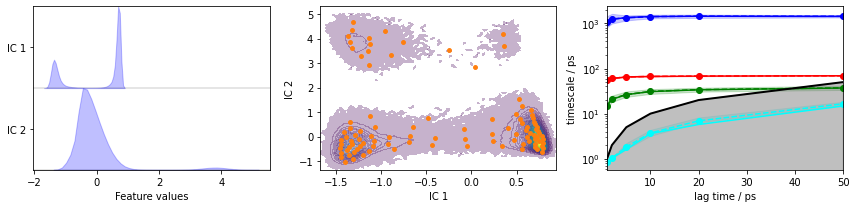### Exercise 2¶

Estimate an MSM at lag time $$10$$ ps with dt_traj='1 ps' and visualize the stationary distribution using a two-dimensional colored scatter plot of all data points in TICA space.

Solution

:

msm = pyemma.msm.estimate_markov_model(cluster.dtrajs, lag=10, dt_traj='1 ps')

print('fraction of states used = {:f}'.format(msm.active_state_fraction))
print('fraction of counts used = {:f}'.format(msm.active_count_fraction))

fig, ax, misc = pyemma.plots.plot_contour(
*tica_concatenated.T, msm.pi[dtrajs_concatenated],
cbar_label='stationary_distribution',
ax.scatter(*cluster.clustercenters.T, s=15, c='C1')
ax.set_xlabel('IC 1')
ax.set_ylabel('IC 2')
fig.tight_layout()

fraction of states used = 1.000000
fraction of counts used = 1.000000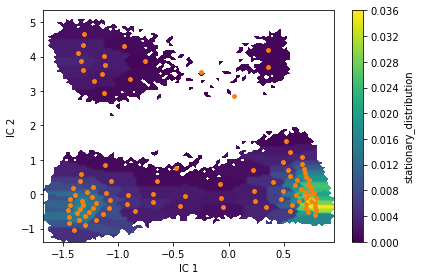### Exercise 3¶

Visualize the first six right eigenvectors.

Solution

:

eigvec = msm.eigenvectors_right()
print('first eigenvector is one: {} (min={}, max={})'.format(
np.allclose(eigvec[:, 0], 1, atol=1e-15), eigvec[:, 0].min(), eigvec[:, 0].max()))

fig, axes = plt.subplots(2, 3, figsize=(12, 6))
for i, ax in enumerate(axes.flat):
pyemma.plots.plot_contour(
*tica_concatenated.T, eigvec[dtrajs_concatenated, i + 1], ax=ax, cmap='PiYG',
cbar_label='{}. right eigenvector'.format(i + 2), mask=True)
ax.scatter(*cluster.clustercenters.T, s=15, c='C1')
ax.set_xlabel('IC 1')
ax.set_ylabel('IC 2')
fig.tight_layout()

first eigenvector is one: True (min=0.999999999999995, max=1.0000000000000668)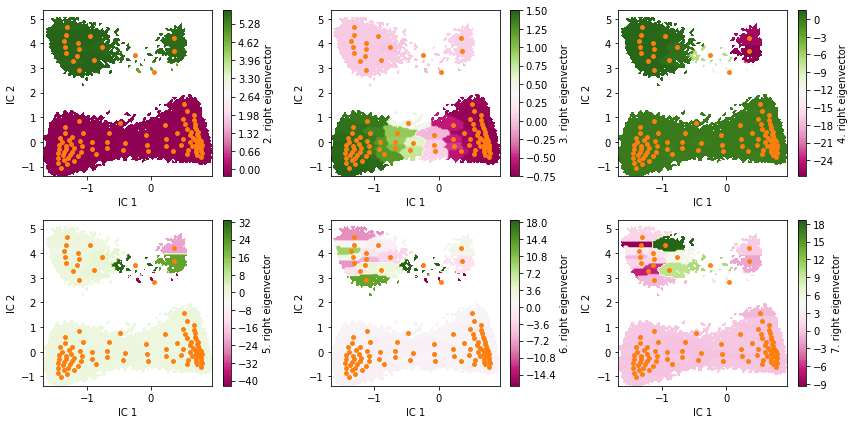Can you already guess from eigenvectors two to four which the metastable states are?

### Exercise 4¶

Estimate a Bayesian MSM at lag time $$10$$ ps and perform/show a CK test for four metastable states.

Solution

:

bayesian_msm = pyemma.msm.bayesian_markov_model(cluster.dtrajs, lag=10, dt_traj='1 ps')

nstates = 4
pyemma.plots.plot_cktest(bayesian_msm.cktest(nstates), units='ps');

:

(<Figure size 720x720 with 16 Axes>,
array([[<AxesSubplot:ylabel='probability'>, <AxesSubplot:>,
<AxesSubplot:>, <AxesSubplot:>],
[<AxesSubplot:ylabel='probability'>, <AxesSubplot:>,
<AxesSubplot:>, <AxesSubplot:>],
[<AxesSubplot:ylabel='probability'>, <AxesSubplot:>,
<AxesSubplot:>, <AxesSubplot:>],
[<AxesSubplot:xlabel='lag time (ps)', ylabel='probability'>,
<AxesSubplot:xlabel='lag time (ps)'>,
<AxesSubplot:xlabel='lag time (ps)'>,
<AxesSubplot:xlabel='lag time (ps)'>]], dtype=object))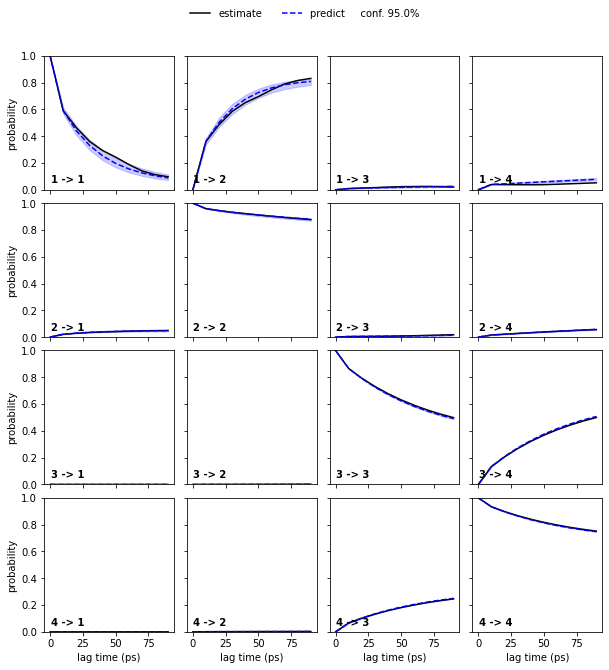### Exercise 5¶

Save the MSM, Bayesian MSM and Cluster objects to the same file as before. Use the model names ala2tica_msm, ala2tica_bayesian_msm and ala2tica_cluster, respectively. Further, include the TICA object with model name ala2tica_tica.

Solution

:

cluster.save('nb4.pyemma', model_name='ala2tica_cluster', overwrite=True)
msm.save('nb4.pyemma', model_name='ala2tica_msm', overwrite=True)
bayesian_msm.save('nb4.pyemma', model_name='ala2tica_bayesian_msm', overwrite=True)
tica.save('nb4.pyemma', model_name='ala2tica_tica', overwrite=True)

---------------------------------------------------------------------------
OSError                                   Traceback (most recent call last)
<ipython-input-1-e53ae0d475a2> in <module>
----> 1 cluster.save('nb4.pyemma', model_name='ala2tica_cluster', overwrite=True)
2 msm.save('nb4.pyemma', model_name='ala2tica_msm', overwrite=True)
3 bayesian_msm.save('nb4.pyemma', model_name='ala2tica_bayesian_msm', overwrite=True)
4 tica.save('nb4.pyemma', model_name='ala2tica_tica', overwrite=True)

/storage/mi/marscher/software/miniconda3/conda-bld/pyemma-doc_1607519340854/_h_env_placehold_placehold_placehold_placehold_placehold_placehold_placehold_placehold_placehold_placehold_placehold_placehold_placehold_placehold_placehold_placehold_placehold_pl/lib/python3.9/site-packages/pyemma/_base/serialization/serialization.py in save(self, file_name, model_name, overwrite, save_streaming_chain)
236         >>> np.testing.assert_equal(m.P, inst_restored.P) # doctest: +SKIP
237         """
--> 238         from pyemma._base.serialization.h5file import H5File
239         try:
240             with H5File(file_name=file_name, mode='a') as f:

/storage/mi/marscher/software/miniconda3/conda-bld/pyemma-doc_1607519340854/_h_env_placehold_placehold_placehold_placehold_placehold_placehold_placehold_placehold_placehold_placehold_placehold_placehold_placehold_placehold_placehold_placehold_placehold_pl/lib/python3.9/site-packages/pyemma/_base/serialization/h5file.py in <module>
20 import numpy as np
21 from io import BytesIO
---> 22 from .pickle_extensions import HDF5PersistentPickler
23
24 __author__ = 'marscher'

/storage/mi/marscher/software/miniconda3/conda-bld/pyemma-doc_1607519340854/_h_env_placehold_placehold_placehold_placehold_placehold_placehold_placehold_placehold_placehold_placehold_placehold_placehold_placehold_placehold_placehold_placehold_placehold_pl/lib/python3.9/site-packages/pyemma/_base/serialization/pickle_extensions.py in <module>
66
67 # we cache this during runtime
---> 68 _DEFAULT_BLOSC_OPTIONS = _check_blosc_avail()
69
70

/storage/mi/marscher/software/miniconda3/conda-bld/pyemma-doc_1607519340854/_h_env_placehold_placehold_placehold_placehold_placehold_placehold_placehold_placehold_placehold_placehold_placehold_placehold_placehold_placehold_placehold_placehold_placehold_pl/lib/python3.9/site-packages/pyemma/_base/serialization/pickle_extensions.py in _check_blosc_avail()
44     fid, name = tempfile.mkstemp()
45     try:
---> 46         with h5py.File(name) as h5f:
47             try:
48                 h5f.create_dataset('test', shape=(1,1), **blosc_opts)

/storage/mi/marscher/software/miniconda3/conda-bld/pyemma-doc_1607519340854/_h_env_placehold_placehold_placehold_placehold_placehold_placehold_placehold_placehold_placehold_placehold_placehold_placehold_placehold_placehold_placehold_placehold_placehold_pl/lib/python3.9/site-packages/h5py/_hl/files.py in __init__(self, name, mode, driver, libver, userblock_size, swmr, rdcc_nslots, rdcc_nbytes, rdcc_w0, track_order, fs_strategy, fs_persist, fs_threshold, **kwds)
422             with phil:
423                 fapl = make_fapl(driver, libver, rdcc_nslots, rdcc_nbytes, rdcc_w0, **kwds)
--> 424                 fid = make_fid(name, mode, userblock_size,
425                                fapl, fcpl=make_fcpl(track_order=track_order, fs_strategy=fs_strategy,
426                                fs_persist=fs_persist, fs_threshold=fs_threshold),

/storage/mi/marscher/software/miniconda3/conda-bld/pyemma-doc_1607519340854/_h_env_placehold_placehold_placehold_placehold_placehold_placehold_placehold_placehold_placehold_placehold_placehold_placehold_placehold_placehold_placehold_placehold_placehold_pl/lib/python3.9/site-packages/h5py/_hl/files.py in make_fid(name, mode, userblock_size, fapl, fcpl, swmr)
188         if swmr and swmr_support:
--> 190         fid = h5f.open(name, flags, fapl=fapl)
191     elif mode == 'r+':
192         fid = h5f.open(name, h5f.ACC_RDWR, fapl=fapl)

h5py/_objects.pyx in h5py._objects.with_phil.wrapper()

h5py/_objects.pyx in h5py._objects.with_phil.wrapper()

h5py/h5f.pyx in h5py.h5f.open()


In this notebook, we have learned how to analyze an MSM and how to extract kinetic information from the model. In detail, we have used - the active_state_fraction, active_count_fraction, and active_set attributes of an MSM object to see how much (and which parts) of our data form the largest connected set represented by the MSM, - the stationary_distribution (or pi) attribute of an MSM object to access its stationary vector, - the eigenvectors_right() method of an MSM object to access its (right) eigenvectors,
For visualizing MSMs or kinetic networks we used - pyemma.plots.plot_density() - pyemma.plots.plot_contour() and - pyemma.plots.plot_cktest().